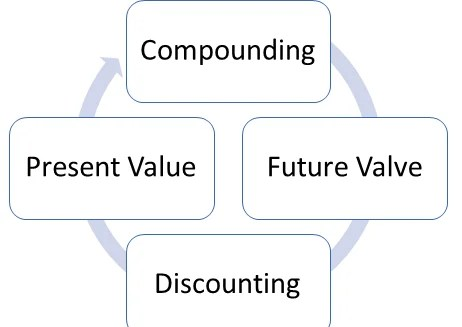# Time Value of Money, Present, Future Value of Money

The time value of money is generally a principle within a financial theory which states that the people if given a preference, would like to receive the money as sooner as possible. It lays the foundation for many of the theories which mainly determines the discount rate that one should expect during the future cash flows.

Time Value of Money says that the worth of a unit of money is going to be changed in future. Put simply, the value of one rupee today will be decreased in future. The whole concept is about the present value and future value of money. There are two methods used for ascertaining the worth of money at different points of time, namely, compounding and discounting. Compounding method is used to know the future value of present money. Conversely, discounting is a way to compute the present value of future money.This principle does not calculate the market value of that asset, instead, it calculates the intrinsic value. Intrinsic value is nothing but the total value that is required to own the asset for the entirety of its lifetime. For better understanding, let’s consider an example. Every business to operate requires machinery for its operations.

The machinery is the necessity for every business and thus whenever the machine or in business terms, assets reaches its life, it needs to replace. Now, the machine that we used to replace the current one reaches its life, also requires to be replaced at the end of that asset’s life. And so the process goes on and on till the business is being run.

This replacement of machines results into the outflow of the cash and thus the whole amount can be added to get a depreciation amount. This is called the depreciation value. There are many methods to calculate this depreciation of any machinery. These methods include the write-down value method, the straight-line method, etc.

 Compounding Discounting Meaning The method used to determine the future value of present investment is known as Compounding. The method used to determine the present value of future cash flows is known as Discounting. Concept If we invest some money today, what will be the amount we get at a future date. What should be the amount we need to invest today, to get a specific amount in future. Use of Compound interest rate. Discount rate Known Present Value Future Value Factor Future Value Factor or Compounding Factor Present Value Factor or Discounting Factor Formula FV = PV (1 + r)^n PV = FV / (1 + r)^n

Assumptions

While talking about the time value of money principle, there are many assumptions that you need to make so that the depreciation can be demonstrated in a simple manner. Some of these assumptions include:

• Each machinery that will be replaced will have the same cost
• The productivity of all the assets remains the same during its lifetime
• The asset and its replacement have the same total lifetime
• There will be no residual value of the asset at the end of its lifetime
• The asset that is used for replacement will have the same productivity
• The assets that are used does not require any repairs or maintenance during its lifetime
• No taxation is involved in the transaction
• The payment done for the asset which is replaced will be upfront
• The replacement of the asset will be immediately done

Present Value and Future Value

While compounding value for the depreciation of the assets, you need to keep in mind two important values: present value and future value. Future value is the value of the asset after a certain time period. While the present value is the value of the asset that we calculate after deducting the residual value.

FV = PV(1 + r)n

where FV= future value,PV = present value, r = rate of interest, n = equal number of periods.

PV = FV / (1 + r)n

Compounding

For understanding the concept of compounding, first of all, you need to know about the term future value. The money you invest today, will grow and earn interest on it, after a certain period, which will automatically change its value in future. So the worth of the investment in future is known as its Future Value. Compounding refers to the process of earning interest on both the principal amount, as well as accrued interest by reinvesting the entire amount to generate more interest.

Compounding is the method used in finding out the future value of the present investment. The future value can be computed by applying the compound interest formula which is as under:Where n = number of years
R = Rate of return on investment.

Discounting

Discounting is the process of converting the future amount into its Present Value. Now you may wonder what is the present value? The current value of the given future value is known as Present Value. The discounting technique helps to ascertain the present value of future cash flows by applying a discount rate. The following formula is used to know the present value of a future sum:Where 1,2,3,…..n represents future years
FV = Cash flows generated in different years,
R = Discount Rate#### intactone

View all posts by intactone →
error: Content is protected !!